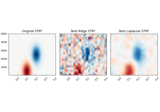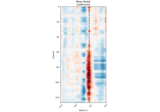# mne.decoding.ReceptiveField#

class mne.decoding.ReceptiveField(tmin, tmax, sfreq, feature_names=None, estimator=None, fit_intercept=None, scoring='r2', patterns=False, n_jobs=None, edge_correction=True, verbose=None)[source]#

Fit a receptive field model.

This allows you to fit an encoding model (stimulus to brain) or a decoding model (brain to stimulus) using time-lagged input features (for example, a spectro- or spatio-temporal receptive field, or STRF) .

Parameters:
tmin`float`

The starting lag, in seconds (or samples if `sfreq` == 1).

tmax`float`

The ending lag, in seconds (or samples if `sfreq` == 1). Must be >= tmin.

sfreq`float`

The sampling frequency used to convert times into samples.

feature_names`array`, shape (n_features,) | `None`

Names for input features to the model. If None, feature names will be auto-generated from the shape of input data after running `fit`.

estimator

The model used in fitting inputs and outputs. This can be any scikit-learn-style model that contains a fit and predict method. If a float is passed, it will be interpreted as the `alpha` parameter to be passed to a Ridge regression model. If `None`, then a Ridge regression model with an alpha of 0 will be used.

fit_intercept

If True (default), the sample mean is removed before fitting. If `estimator` is a `sklearn.base.BaseEstimator`, this must be None or match `estimator.fit_intercept`.

scoring[‘r2’, ‘corrcoef’]

Defines how predictions will be scored. Currently must be one of ‘r2’ (coefficient of determination) or ‘corrcoef’ (the correlation coefficient).

patterns`bool`

If True, inverse coefficients will be computed upon fitting using the covariance matrix of the inputs, and the cross-covariance of the inputs/outputs, according to . Defaults to False.

n_jobs

Number of jobs to run in parallel. Can be ‘cuda’ if CuPy is installed properly and `estimator is None`.

New in version 0.18.

edge_correction`bool`

If True (default), correct the autocorrelation coefficients for non-zero delays for the fact that fewer samples are available. Disabling this speeds up performance at the cost of accuracy depending on the relationship between epoch length and model duration. Only used if `estimator` is float or None.

New in version 0.18.

verbose

Control verbosity of the logging output. If `None`, use the default verbosity level. See the logging documentation and `mne.verbose()` for details. Should only be passed as a keyword argument.

Notes

For a causal system, the encoding model will have significant non-zero values only at positive lags. In other words, lags point backward in time relative to the input, so positive lags correspond to previous input time samples, while negative lags correspond to future input time samples.

References

Attributes:
coef_array, shape ([n_outputs, ]n_features, n_delays)

The coefficients from the model fit, reshaped for easy visualization. During `mne.decoding.ReceptiveField.fit()`, if `y` has one dimension (time), the `n_outputs` dimension here is omitted.

patterns_array, shape ([n_outputs, ]n_features, n_delays)

If fit, the inverted coefficients from the model.

delays_`array`, shape (n_delays,), dtype `int`

The delays used to fit the model, in indices. To return the delays in seconds, use `self.delays_ / self.sfreq`

valid_samples_`slice`

The rows to keep during model fitting after removing rows with missing values due to time delaying. This can be used to get an output equivalent to using `numpy.convolve()` or `numpy.correlate()` with `mode='valid'`.

Methods

 `fit`(X, y) Fit a receptive field model. `get_params`([deep]) Get parameters for this estimator. Generate predictions with a receptive field. `score`(X, y) Score predictions generated with a receptive field. `set_params`(**params) Set the parameters of this estimator.
fit(X, y)[source]#

Fit a receptive field model.

Parameters:
X`array`, shape (n_times[, n_epochs], n_features)

The input features for the model.

y`array`, shape (n_times[, n_epochs][, n_outputs])

The output features for the model.

Returns:
selfinstance

The instance so you can chain operations.

Examples using `fit`:Spectro-temporal receptive field (STRF) estimation on continuous data

Spectro-temporal receptive field (STRF) estimation on continuous dataReceptive Field Estimation and Prediction

Receptive Field Estimation and Prediction
get_params(deep=True)[source]#

Get parameters for this estimator.

Parameters:
deep`bool`, optional

If True, will return the parameters for this estimator and contained subobjects that are estimators.

Returns:
params`dict`

Parameter names mapped to their values.

predict(X)[source]#

Generate predictions with a receptive field.

Parameters:
X`array`, shape (n_times[, n_epochs], n_channels)

The input features for the model.

Returns:
y_pred`array`, shape (n_times[, n_epochs][, n_outputs])

The output predictions. “Note that valid samples (those unaffected by edge artifacts during the time delaying step) can be obtained using `y_pred[rf.valid_samples_]`.

Examples using `predict`:Spectro-temporal receptive field (STRF) estimation on continuous data

Spectro-temporal receptive field (STRF) estimation on continuous dataReceptive Field Estimation and Prediction

Receptive Field Estimation and Prediction
score(X, y)[source]#

Score predictions generated with a receptive field.

This calls `self.predict`, then masks the output of this and `y` with ``self.valid_samples_`. Finally, it passes this to a `sklearn.metrics` scorer.

Parameters:
X`array`, shape (n_times[, n_epochs], n_channels)

The input features for the model.

y`array`, shape (n_times[, n_epochs][, n_outputs])

Used for scikit-learn compatibility.

Returns:
scores`list` of `float`, shape (n_outputs,)

The scores estimated by the model for each output (e.g. mean R2 of `predict(X)`).

Examples using `score`:Spectro-temporal receptive field (STRF) estimation on continuous data

Spectro-temporal receptive field (STRF) estimation on continuous dataReceptive Field Estimation and Prediction

Receptive Field Estimation and Prediction
set_params(**params)[source]#

Set the parameters of this estimator.

The method works on simple estimators as well as on nested objects (such as pipelines). The latter have parameters of the form `<component>__<parameter>` so that it’s possible to update each component of a nested object.

Parameters:
**params`dict`

Parameters.

Returns:
instinstance

The object.

## Examples using `mne.decoding.ReceptiveField`#Spectro-temporal receptive field (STRF) estimation on continuous data

Spectro-temporal receptive field (STRF) estimation on continuous dataReceptive Field Estimation and Prediction

Receptive Field Estimation and Prediction